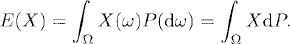# EXPECTATION

In probability theory, the expectation of a random variable is the integral of the random variable with respect to its probability measure. For example, the expectation of a discrete random variable is the weighted average of all possible values that the random variable can take on. In this chapter, we shall introduce the definition of expectation and relevant concepts.

# 13.1 Basic Concepts and Facts

Definition 13.1 (Expectation). Let (Ω,, P) be a probability space and XL1 (Ω,, P), where L1(Ω,, P) denotes the set of all P-integrable functions on Ω, (see Definition 6.4). The expectation E(X) of X is defined asDefinition 13.2 (Expectation over Subsets). Let (Ω,, P) be a probability space, F, and X be a random variable on Ω. Then E(X; F) is defined as

where

Definition ...

Get Measure, Probability, and Mathematical Finance: A Problem-Oriented Approach now with the O’Reilly learning platform.

O’Reilly members experience live online training, plus books, videos, and digital content from nearly 200 publishers.